# A Gallery of Spectral Correlation

In this post I provide plots of the spectral correlation for a variety of simulated textbook signals and several collected communication signals. The plots show the variety of cycle-frequency patterns that arise from the disparate approaches to digital communication signaling. The distinguishability of these patterns, combined with the inability to distinguish based on the power spectrum, leads to a powerful set of classification (modulation recognition) features (My Papers [16, 25, 26, 28]).

In all cases, the cycle frequencies are blindly estimated by the strip spectral correlation analyzer (The Literature [R3, R4]) and the estimates used by the FSM to compute the spectral correlation function. MATLAB is then used to plot the magnitude of the spectral correlation and conjugate spectral correlation, as specified by the determined non-conjugate and conjugate cycle frequencies.

There are three categories of signal types in this gallery: textbook signals, collected signals, and feature-rich signals. The latter comprises some collected signals (e.g., LTE) and some simulated radar signals. For the first two signal categories, the three-dimensional surface plots I’ve been using will suffice for illustrating the cycle-frequency patterns and the behavior of the spectral correlation function over frequency. But for the last category, the number of cycle frequencies is so large that the three-dimensional surface is difficult to interpret–it is a visual mess. For these signals, I’ll plot the maximum spectral correlation magnitude over spectral frequency$f$ versus the detected cycle frequency$\alpha$ (as in this post).

A complementary gallery of cyclic autocorrelation functions can be found here.

### Textbook Signals (Simulated)

For the textbook signals the sampling rate is set to$1.0$. Multiply the$f$ and$\alpha$ axes by any sampling rate of interest to see the actual spectral widths of the features. For those signals with a symbol rate, it is set to$0.1$, and for all signals the carrier frequency is set to$0.05$. The FSM is used to estimate the spectral correlation function for each of the obtained cycle frequencies. The number of samples (block length) used by the FSM is$65536$ and the width of the frequency smoothing window is$0.025$ ($2.5$% of the sampling rate).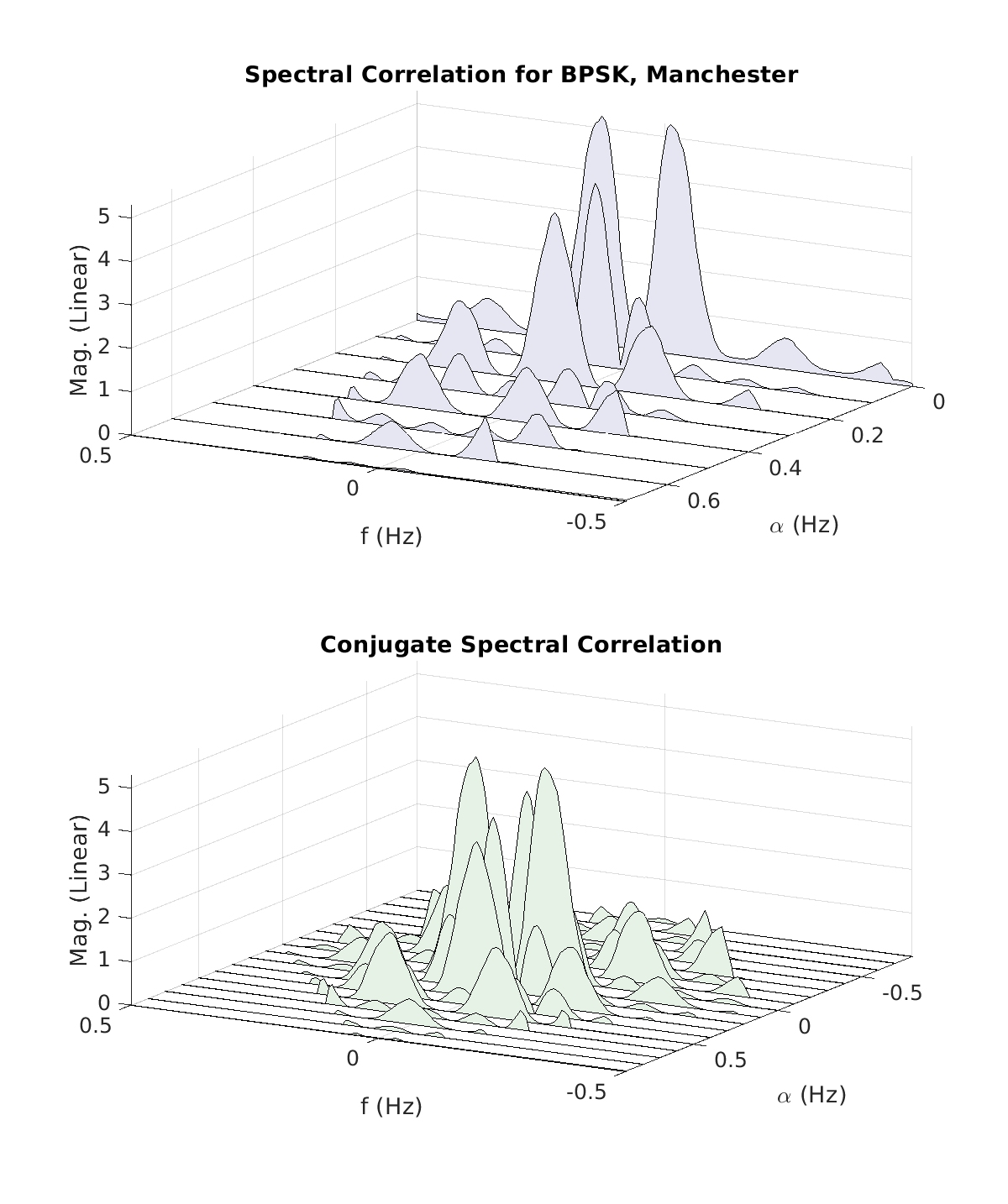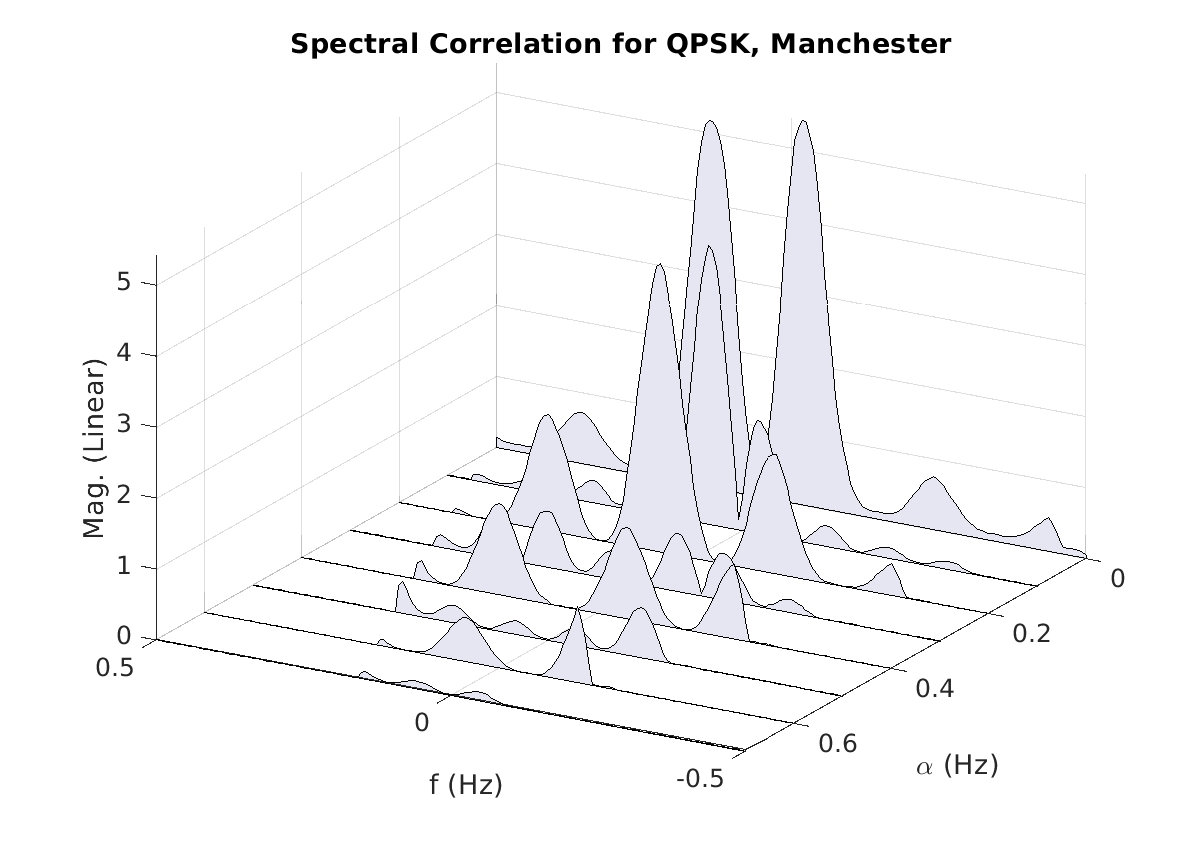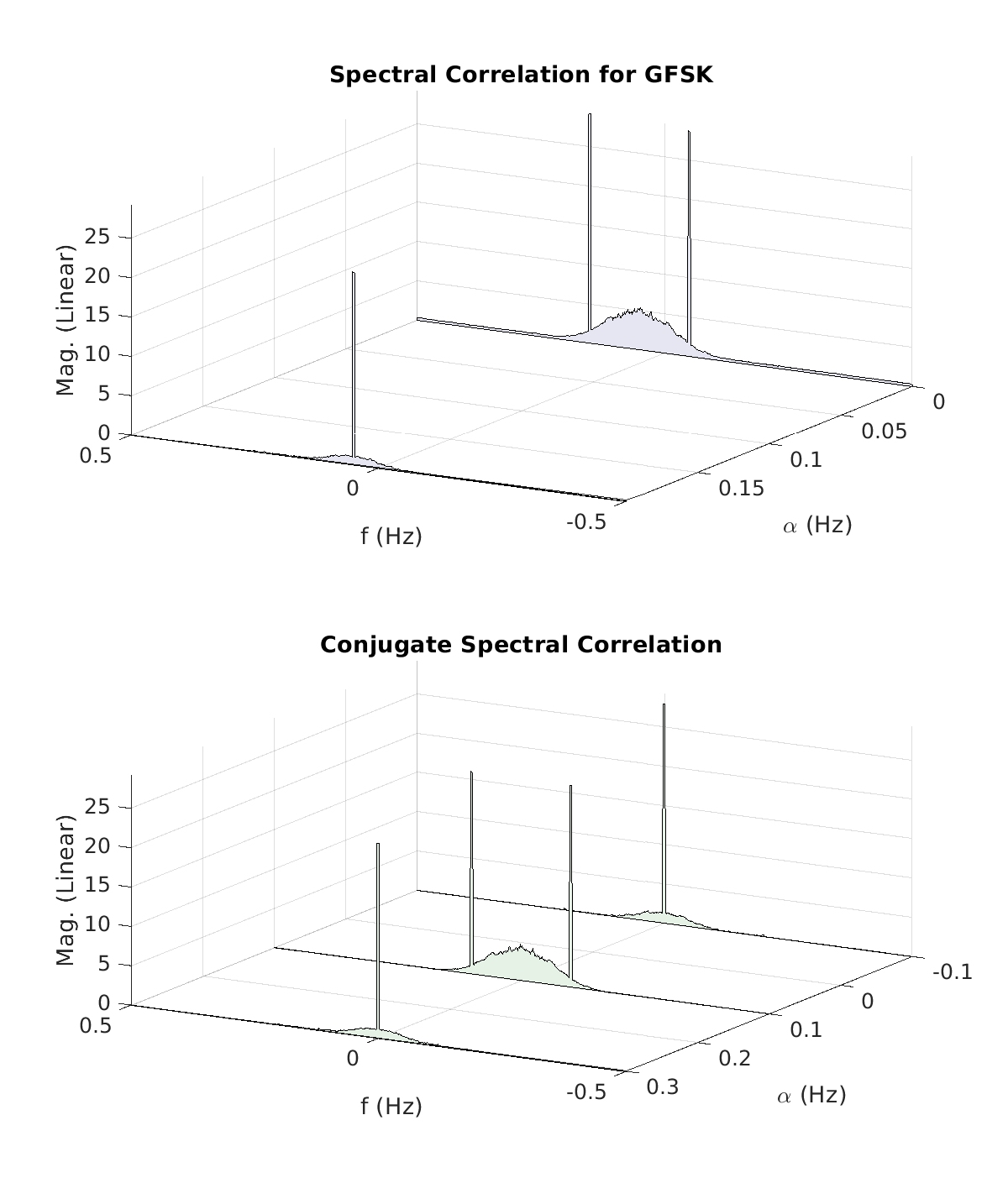A key aspect of the textbook gallery is that some distinct signal types give rise to identical spectral correlation functions. In particular, the spectral correlation functions for rectangular-pulse QPSK, 8PSK, 16QAM, and$\pi/4$-DQPSK are identical. Similarly, the spectral correlation functions for square-root raised-cosine (SRRC) QPSK, 8PSK, 16QAM, and$\pi/4$-DQPSK are identical, because they all use the same roll-off factor of$1.0$. The higher-order statistics for these signals are, fortunately, not identical.

### Collected Signals

The measured spectral correlation functions for four collected (captured over the air) communication signals are shown here:

The cycle frequencies for WCDMA (downlink) include the chip rate$3.84$ MHz as well as$120.0$ kHz and$15.0$ kHz. For CDMA EVDO (downlink), we have the CDMA chip rate of$1.2288$ MHz as well as several harmonics of$1.230$ MHz. For CDMA (downlink) we have the chip rate$1.2288$ MHz, and for ATSC Digital TV, we have the two conjugate cycle frequencies equal to$2f_c \pm 10.7622e6/2$. See My Papers  for more details on the cyclostationarity of these and other real-world signals. Notice that the PSDs for the four collected signals show the effects of a multipath propagation channel between the transmitter and my lab. The multipath channel distorts both the spectrum and the spectral correlation function, but does not modify the values of the cycle frequencies.

### Feature-Rich Signals

Finally, the following sequence of plots shows the spectral correlation for a few signal types possessing a great many cycle frequencies. The usual way of plotting the spectral correlation function is not helpful here as our eyes cannot untangle the many individual features in the hidden-line display.

The two radar signals are simulated. The LFM radar signal is simply a train of identical linear FM pulses (chirps), whereas the phase-coded radar signal is a train of identical PSK-like signals employing a binary minimum peak sidelobe sequence. The pulse repetition interval for both is$4096$ samples (sampling rate is$1.0$ for the radar signals). The duty cycles are about$0.03$. These signals are periodic, and so are in a sense trivially second-order cyclostationary signals. This is because they consist of a sum of finite-strength sine-wave components, and so naturally there are finite-strength sine-wave components in second- and higher-order nonlinear transformations of the signal.

The LTE signals produce nearly as many cycle frequencies as the radar signals, but their cyclostationarity is not trivial. That is, the LTE signals are not periodic. But they are fiendishly complex, and this complexity leads to a complex cyclostationarity. Finally, the GSM signal is also quite complex relative to its base modulation of GMSK, so we see the appearance of many cycle frequencies separated by a harmonic of the frame rate, which is$216.7$ Hz.

The signal files I used to create these estimates are available on my Downloads page.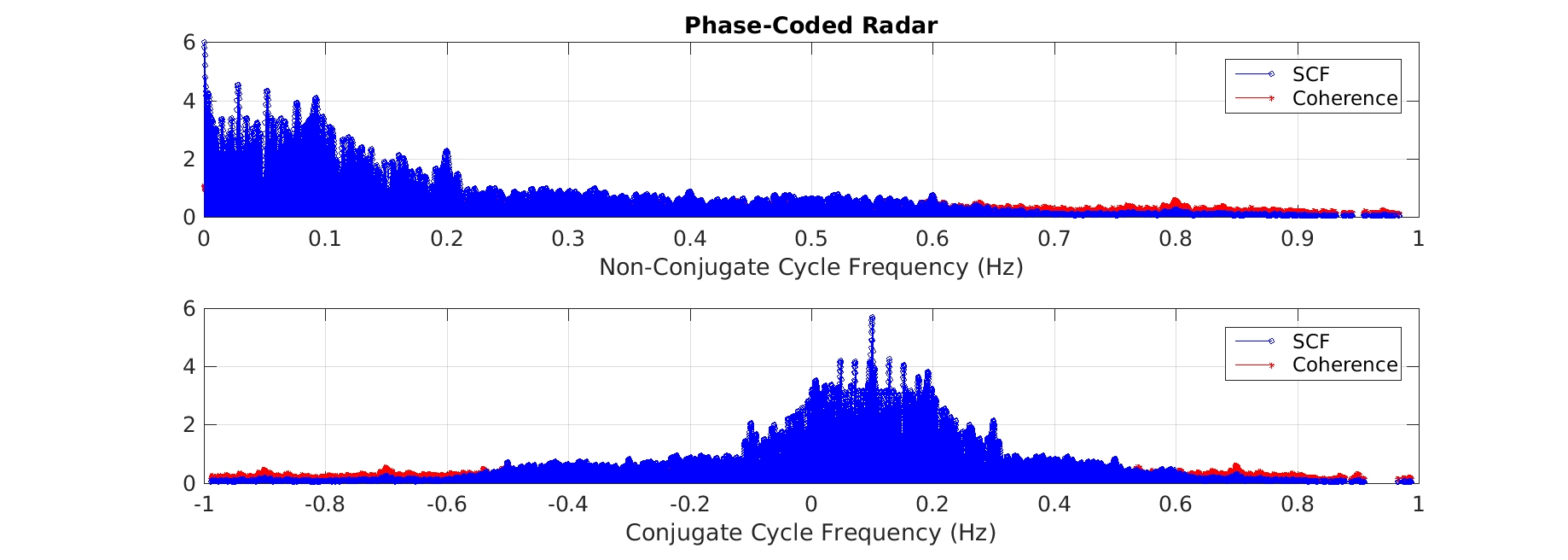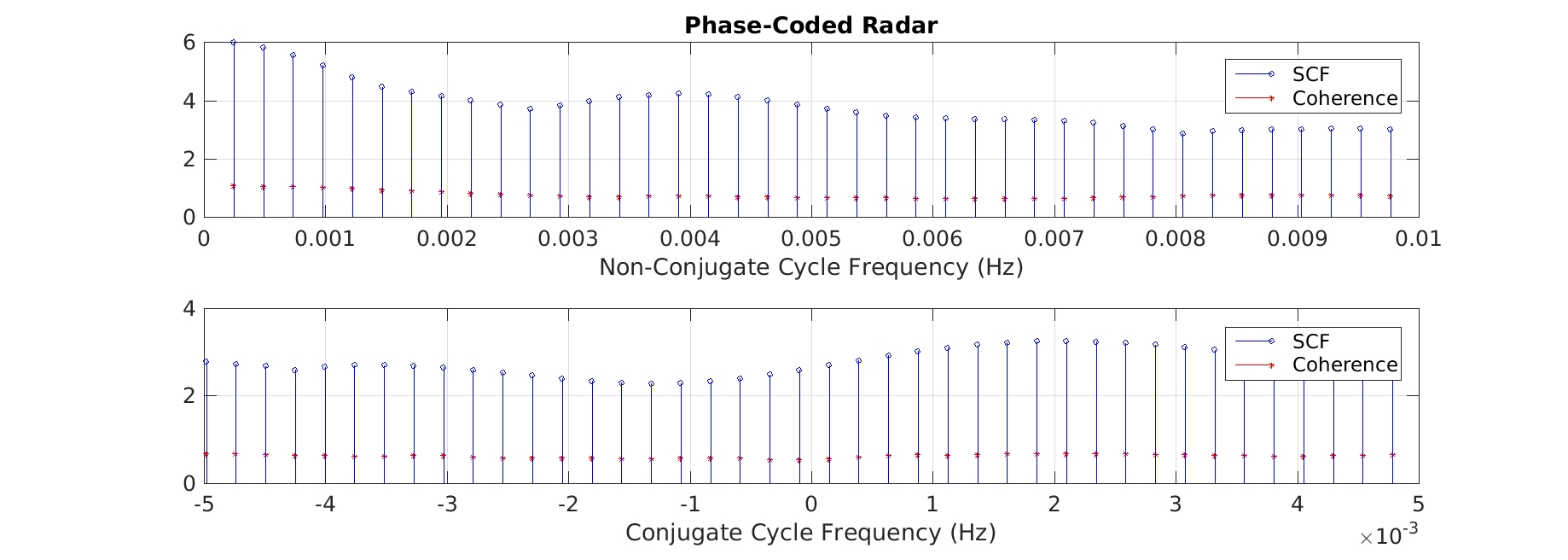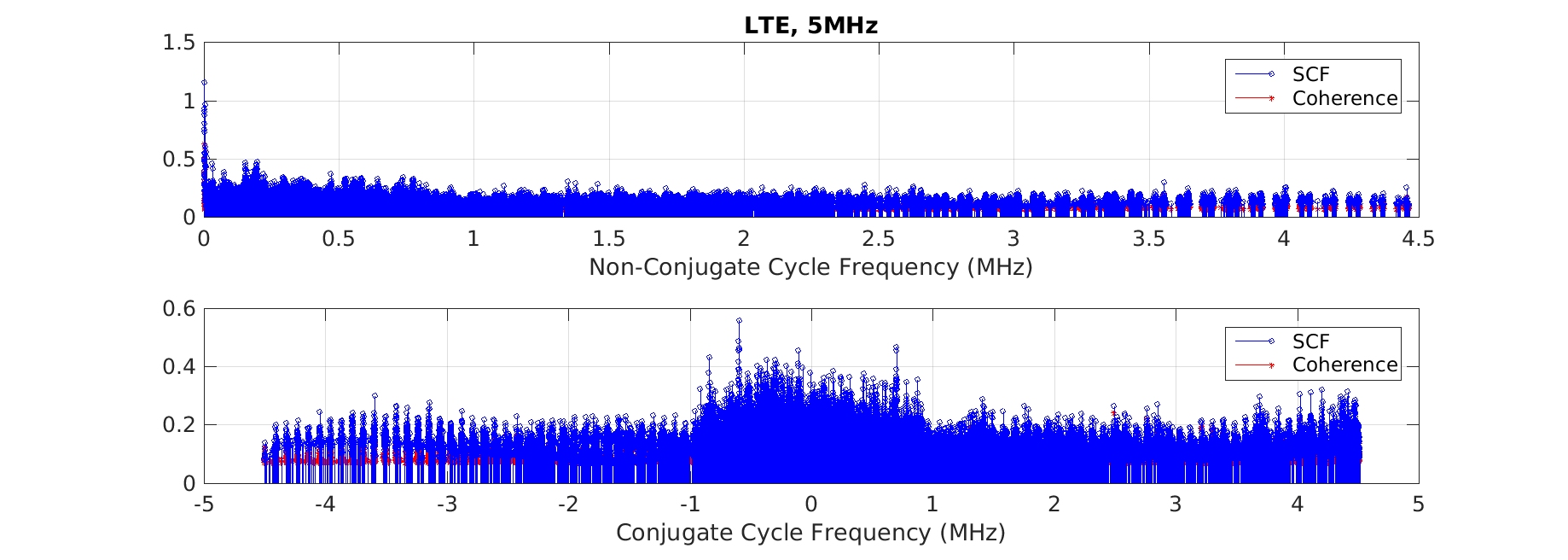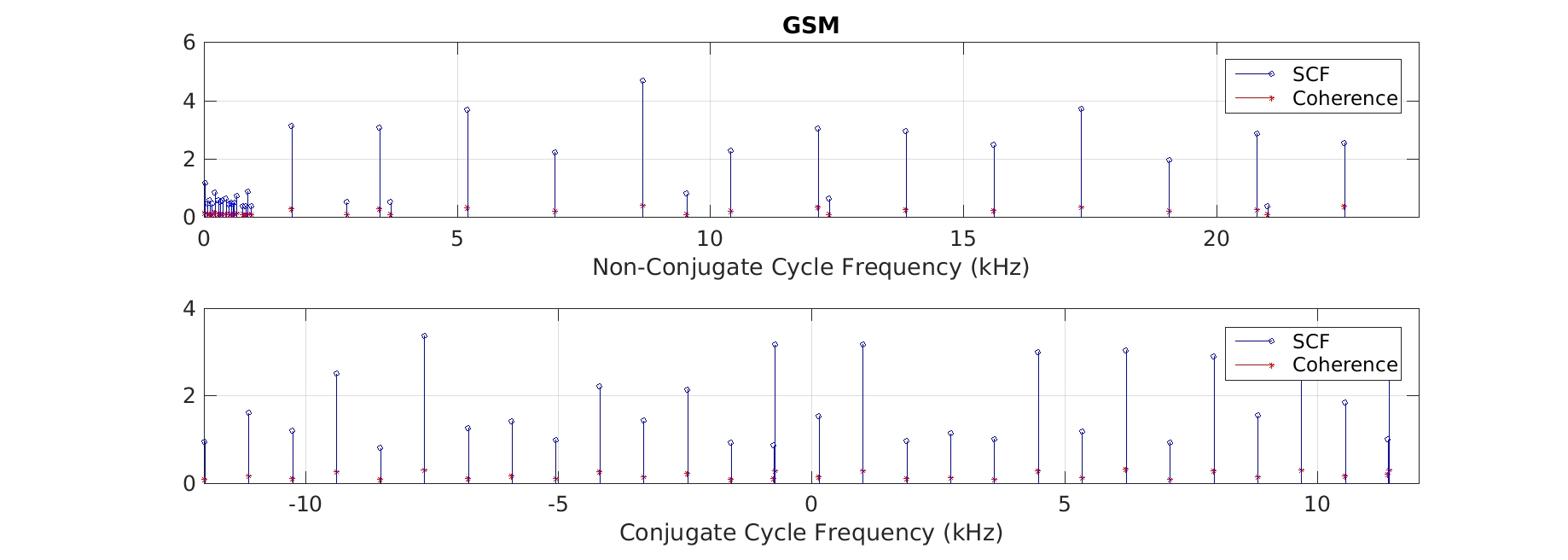## 15 thoughts on “A Gallery of Spectral Correlation”

1.Mohamed says:

Hi Chad, what is the unique about feature-rich signals, I mean what make them contains that many cyclic frequencies? for example, is it due to the modulation or coding types they are using?

•Chad Spooner says:

Yes, it is due to the specific nature of the modulation. In particular, if a signal combines a high-rate modulation type with one or more low-rate medium-access control processes, these can mix and you can end up with a large number of harmonically related cycle frequencies. The simpler the modulation (think textbook signals), the simpler the cyclostationarity as well.

2.Chen says:

Hi Chad
Could you please provide the simulation parameters and theoretical spectral correlation surfaces (three dimension surfaces) for DSSS-BPSK and DSSS-QPSK? I have refered to the book, “Statistical Spectral Analysis A Nonprobabilistic Theory”, which provided spectral correlation surfaces for DSSS-QPSK. There is a little bit difference between the results in that book and yours, namely simulation results of spectral correlation surfaces for DSSS-QPSK. Why?

•Chad Spooner says:

Have you studied my post on DSSS signals? The short answer to your question is that there is no single DSSS QPSK or DSSS BPSK signal. There are many variants involving the choices of different parameters (code class, code length, modulation type, pulse-shaping function, etc.).